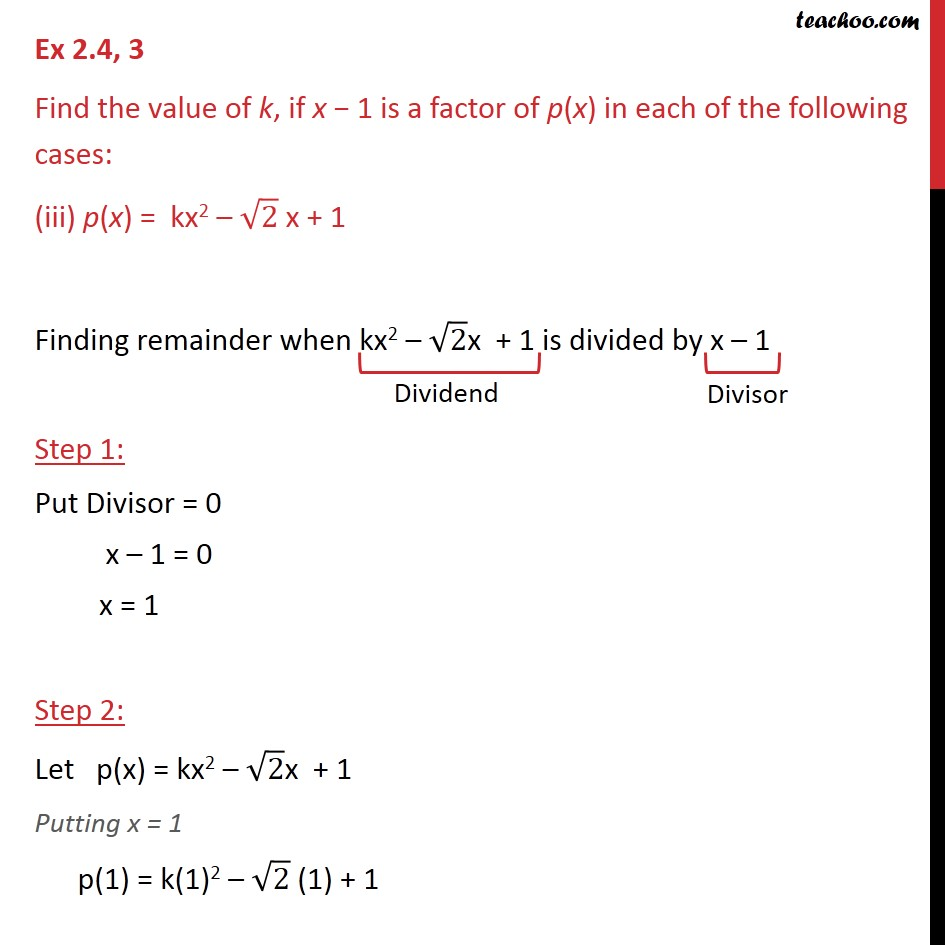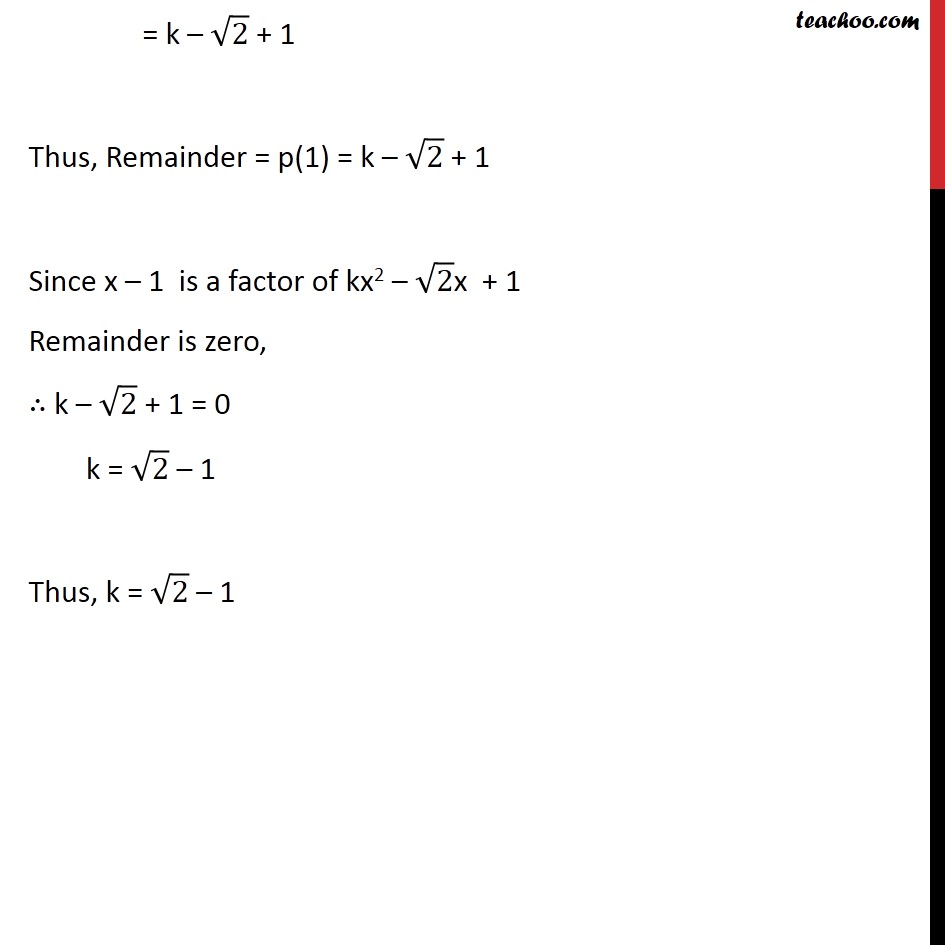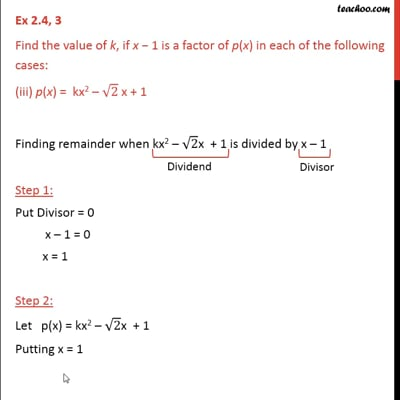Ex 2.4

Chapter 2 Class 9 Polynomials
Serial order wiseThis video is only available for Teachoo black users

Maths Crash Course - Live lectures + all videos + Real time Doubt solving!

### Transcript

Ex 2.4, 3 Find the value of k, if x 1 is a factor of p(x) in each of the following cases: (iii) p(x) = kx2 2 x + 1 Finding remainder when kx2 2x + 1 is divided by x 1 Step 1: Put Divisor = 0 x 1 = 0 x = 1 Step 2: Let p(x) = kx2 2x + 1 Putting x = 1 p(1) = k(1)2 2 (1) + 1 = k 2 + 1 Thus, Remainder = p(1) = k 2 + 1 Since x 1 is a factor of kx2 2x + 1 Remainder is zero, k 2 + 1 = 0 k = 2 1 Thus, k = 2 1